# Arithmetic Progressions Class 10 Extra Questions Maths Chapter 5 with Solutions Answers

Here you will find Arithmetic Progressions Class 10 Extra Questions Maths Chapter 5 with Answers Solutions, Extra Questions for Class 10 Maths are solved by experts and will guide students in the right direction.

## Extra Questions for Class 10 Maths Arithmetic Progressions with Answers Solutions

Extra Questions for Class 10 Maths Chapter 5 Arithmetic Progressions with Solutions Answers

### Arithmetic Progressions Class 10 Extra Questions Objective Type

Question 1.
Common difference for arithmetic progression (A.P) 3, 1, -1, -3 ….. will be:
(a) 1
(b) 2
(c) -2
(d) 3
(c) -2
Solution.
Common difference = a2 – a1
= 1 – 3 = -2
Hence, choice (iii) is correct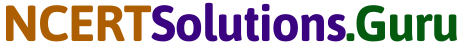Question 2.
Common difference of 2, 0.5, -1, -2.5, -4,….. is:
(a) -1.5
(b) -1.3
(c) 2.4
(d) 2.6
(a) -1.5

Question 3.
100th term of $$\frac {1}{2}$$, 1, $$\frac {3}{2}$$, 2, ….is:
(a) 50
(b) 60
(c) 70
(d) 40
(a) 50

Question 4.
rth term of a + 2b, a – b, a – 46, … is:
(a) a + (5 – 3r)b
(b) a + (4 – 3r)b
(c) a + (6 – r)b
(d) a + (2 – r)b
(a) a + (5 – 3r)b

Question 5.
The sum of first 16 terms of the AP 10, 6, 2, …. is:
(a) 320
(b) -320
(c) -352
(d) -400
(b) -320Question 6.
The sum of first 20 terms of the AP 1, 3, 5, 7, 9, … is
(a) 264
(b) 400
(c) 472
(d) 563
(b) 400

Question 7.
(5 + 13 + 21 + … + 181) =?
(a) 2476
(b) 2337
(c) 2219
(d) 2139
(d) 2139

Question 8.
The sum of n terms of the AP √2, √8, √18, √32, … is
(a) 1
(b) 2n(n + 1)
(c) $$\frac {1}{2}$$n(n + 1)
(d) $$\frac{1}{\sqrt{2}}$$n(n + 1).
(d) $$\frac{1}{\sqrt{2}}$$n(n + 1)

Question 9.
How many terms of the AP 3, 7, 11, 15, ….will make the sum 406?
(a) 10
(b) 12
(c) 14
(d) 20
(c) 14Question 10.
The sum of first 100 natural numbers is
(a) 4950
(b) 5050
(c) 5000
(d) 5150
(b) 5050

Question 11.
The sum of all odd numbers between 100 and 200 is
(a) 3750
(b) 6200
(c) 6500
(d) 7500
(d) 7500

Question 12.
The sum of all even natural numbers less than 100 is
(a) 2272
(b) 2352
(c) 2450
(d) 2468
(c) 2450

Question 13.
The sum of first fifteen multiples of 8 is
(a) 840
(b) 960
(c) 872
(d) 1080
(b) 960Question 14.
In an AP, the first term is 22, nth term is 11 and the sum of first n terms is 66. The value of n is
(a) 10
(b) 12
(c) 14
(d) 16
(b) 12

Question 15.
In an AP, the first term is 8, nth term is 33 and the sum of first n terms is 123. Then, d =?
(a) 5
(b) – 5
(c) 7
(d) 3
(a) 5

Question 16.
The sum of n terms of an AP is given by Sn = (2n2 + 3n). What is the common difference of the AP?
(a) 3
(b) 4
(c) 5
(d) 9
(b) 4

### Arithmetic Progressions Class 10 Extra Questions Very Short answer Type

Question 1.
For the following APs, write the first term and the common difference.
(i) 3, 1, -1, -3, …
(ii) – 5, -1, 3, 7, …..,
(iii) $$\frac{1}{3}, \frac{5}{3}, \frac{9}{3}, \frac{13}{3}$$ ,…..
(iv) 0.6, 1.7, 2.8, 3.9, …
Solution.
(i) First term a = t1 = 3, Common difference
d = 2nd term – 1st term
= 1 – 3 = -2

(ii) First term a = t1 = -5, Common difference
d = 2nd term – 1st term
= -1 -(-5) = 1 + 5 = 4

(iii) First term a = t1 = $$\frac {1}{3}$$ Common difference
d = 2nd term – 1st term = $$\frac{5}{3}-\frac{1}{3}=\frac{4}{3}$$

(iv) First term a = t1 = 0.6 Common difference
d = 2nd term – 1st term
= 1.7 – 0.6 = 1.1Question 2.
For what value of k will k + 9, 2k – 1, and 2k + 7 are the consecutive terms of an arithmetic progression (A.P)
Solution.
Given, k + 9, 2k – 1 and 2k + 7 are the consecutive term
∴ d = a2 – a1 = a3 – a2
⇒ (2k – 1) – (k + 9) = (2k + 7) – (2k – 1)
⇒ 2k – 1 – k – 9 = 2k + 7 – 2k + 1
k – 10 = 8
∴ k = 8 + 10 = 18

Question 3.
For what value of k, will the points (k, -1), (2, 1) and (4, 5) lie on a line.
Solution.
If point (k, -1), (2, 1) and (4, 5) lie on a line, i.e., the points are collinear.
x1 = k, y1 = -1, x2 = 2, y2 = 1, x3 = 4, y3 = 5
The area of A formed by these points are zero.
∴ 0 = $$\frac {1}{2}$$ [x1 (y2 – y3) + x2(y3 – y1) + x3(y1 – y2)]
⇒ k(1 -5) + 2(5 + 1) + 4 (-1 -1) = 0
⇒ -4k + 12 – 8 = 0
⇒ -4k = -4
⇒ k = 1Question 4.
Find the 31st term of an AP whose 11th term is 38 and the 16th term is 73.
Solution.
let a be the first term and d the common difference of an AP. Now, the nth term of an AP is
an = a + (n – 1)d
a11 = a + 10d = 38
[∵ a11 = 38 (given)] …(i)
and a16 = a + 15d = 73
[∵ a16 = 73 (given)] ..(ii)
On subtracting Eq. (i) from EQuestion (ii) we get
5d = 35 = d = $$\frac {35}{5}$$ = 7
From Eq. (i)
a + 10 × 7 = 38
⇒ a = 38 – 70 = – 32
∴ The 31st term of an AP
a31 = a + 30d
= -32 + 30 × 7
= – 32 + 210 = 178Question 5.
Find the sum of the following AP’s
(i) 2, 7, 12, …, to 10 terms
(ii) -37, -33, -29, …, to 12 terms
(iii) 0.6, 1.7, 2.8, … to 100 terms
(iv) $$\frac{1}{15}, \frac{1}{12}, \frac{1}{10}$$ ……., to 11 terms.
Solution.
(i) Let a be the first term and d be the common difference of the given AP.
Then, we have a = 2 and d = 7 – 2 = 5
∵ Sum of n terms of AP,
Sn = $$\frac {n}{2}$$[2a + (n – 1)d]
Putting a = 2, d = 5, n = 10, we get
S10 = $$\frac {10}{2}$$[2 × 2 + (10 – 1)5]
= 5 (4 + 9 × 5)
= 5 (4 + 45) = 5 × 49 = 245
(ii) let a be the first and d be the common difference of the given AP,
Then, we have a = -37,
d = -33 -(-37) = – 33 + 37
= 4
∵ Sum of n terms of an AP,
Sn = $$\frac {n}{2}$$[2a + (n – 1)d], we get
S12 = [2 × (-37) + (12 – 1)4]
= 6(-74 + 11 × 4)
= 6 – 74 +44)
= 6 × (-30) = – 180.
(iii) Let a be the first term and d be the common difference of the given AP.
Then, we have a = 0.6, d = 1.7 -0.6 = 1.1
∵ Sum of n terms of an AP,
Sn = $$\frac {n}{2}$$[2a + (n – 1)d], we get
S100 = $$\frac {100}{2}$$ [2 × 0.6 + (100 – 1) 1.1]
= 50 (1.2 + 99 × 1.1)
= 50 (1.2 + 108.9)
= 50 × 110.1
= 5505
(iv) Let a be the first term and d be the common difference of the given AP.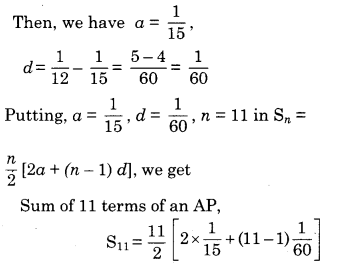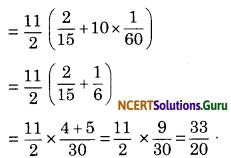Question 6.
The first term of an AP is 5, the last term is 45 and the sum is 400. Find the number of terms and the common difference.
Solution.
Let a be the first term and d be the common difference of an AP.
Give, first term a = 5, last term l = 45 and sum of n terms Sn = 400∴ The number of terms is 16 and the common difference is $$\frac {8}{3}$$.

Question 7.
The first and the last terms of an AP are 17 and 350, respectively. If the common difference is 9, how many terms are there and what is their sum?
Solution.
Let a be the first term and d be the common difference.
Given, first term a = 17, last term l = an = 350, common difference d = 9
∵ l = an = 350
⇒ a + (n – 1)d = 350
[∵ l = an = a + (n – 1)d]
⇒ 17 + (n – 1)9 = 350 =
⇒ 9(n – 1) = 350 – 17 = 333
⇒ n – 1 = $$\frac {333}{9}$$ = 37
⇒ n = 37 + 1 = 38
Putting a = 17,1 = 350, n = 38
∵ Sum of n terms
Sn = $$\frac {n}{2}$$(a + l), we get
S39 = $$\frac {38}{2}$$ (17 + 350)
= 19 (367) = 6973
So, there are 38 terms in the AP having their sum as 6973.Question 8.
Which term of the AP 3, 8, 13, 18, …. is 78?
Solution.
Let nth term, be 78. Given, 3, 8, 13, 18 …. are in AP.
First term a, = 3, common difference, d = 8 – 3 = 5
∵ nth term an = 78
∴ a + (n – 1) )d = 78
⇒ 3 + (n – 1) 5 = 78
⇒ (n – 1) 5 = 78 – 3 5
⇒ (n – 1) = 75 n – 1 = 15
⇒ n = 15 + 1
⇒ n = 16
Hence, 16th term be 78.

Question 9.
Find the number of terms in each of the following APs
(i) 7, 13, 19, …., 205
(ii) 18, 15 $$\frac {1}{2}$$, 13, …., -47
Solution.
(i) Suppose, there are n terms in the given AP. Then nth term an = 205, first term a = 7, common difference d = 13 – 7 = 6.
∵ a + (n – 1) d = an
∴ a + (n – 1)d = 205
⇒ 7 + (n – 1)6 = 205
⇒ 6(n – 1) = 205 – 7
⇒ 6(n – 1) = 198
⇒ n – 1 = $$\frac {198}{6}$$ = 33
n = 33 + 1 = 34
⇒ Hence, the given AP contains 34 terms.

(ii) Suppose, there are n terms in the given AP
Given, first term a = 18, common difference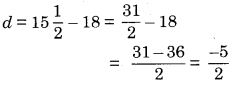Then nth term an = -47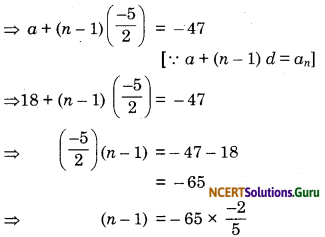= -13 × -2 = 26
⇒ n = 26 + 1 = 27
Hence, the given AP contains 27 terms.

### Arithmetic Progressions Class 10 Extra Questions Short answer Type

Question 1.
Find the sum of all odd integers between z and 100 which are divisible by 3.
Solution.
The odd numbers which are divisible by 3, between 2 and 100 are 3, 9, 15, 21, 27, 33,…..99 which clearly form on A.P. here a = 3, d = 6 and l = 99
∴ 99 = a + (n – 1)d
⇒ 99 = 3 + (n – 1)d
⇒ (n – 1)6 = 99 – 3 = 96
⇒ n = $$\frac {96}{6}$$ + 1 = 17
Now, to find the sum of 17 terms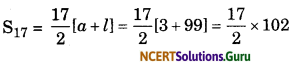= 17 × 51
= 867.Question 2.
Which term of arithmetic progression 21, 18, 15…. is – 81? Is zero any term of this arithmetic progress?
Solution.
Given AP be 21, 18, 15…… Let nth term of this progression is – 81
a = 21, d = -3
∴ -81 = 21 + (n – 1) × -3
⇒ -81 – 21 = -3(n – 1)
⇒ n – 1 = $$\frac {102}{3}$$ = 34
∴ n = 34 + 1
= 35
Hence 35th term of this progression is 81
Let Tn = 0 = 21 + (n – 1)(-3)
⇒ 21 – 3n + 3 = 0
∴ 3n = 24
n = 8
then a 8th term of the progression is 0.

Question 3.
How many terms should taken from the series 9, 17, 25, 33… to get a total of 636?
Solution.
The given series an A.P. where a = 9, d 25 – 17 = 8 and Sn = 636.
⇒ Sn $$\frac {n}{2}$$[2a + (n – 1)d]
⇒ 636 = $$\frac {n}{2}$$[2 × 9 + (n – 1) 8]
⇒ 636 = n [9 + 4n – 4]
⇒ 4n2 + 5n – 636 = 0
⇒ 4n2 + (53 – 48) n – 636 = 0
⇒ 4n2 + 53n – 48n – 636 = 0
⇒ n(4n + 53)- 12 (4n + 53) = 0
⇒ (4n + 53) (n – 12) = 0
∴ n = 12 or – $$\frac {53}{4}$$
as number of terms are not infraction and – ve
∴ n = 12
Hence sum of 12 term of the series = 636.Question 4.
A contract on construction job specifies a penalty for delay of completion beyond a certain date as follows ₹ 200 for the first day, ₹ 250 for the second day, ₹ 300 for the third day etc., the penalty for each succeeding day being ₹ 50 more than for the preceding day. How much money the contractor has to pay as penalty, if he has delayed the work by 30 days?
Solution.
Since, the penalty for each succeeding day is 50 more than the preceding day, therefore the penalties for the first day, the second day, the third day, etc. will form an AP.
Let us denote the penalty for the nth day by an, then
a1 = 200, a2 = ₹ 250, a3 = ₹ 300
Here, a = ₹ 200, d = ₹ 250 – ₹ 200 = ₹ 50 and n = 30.
∴ The money the contractor has to pay penalty, if he delayed the work by 30 days.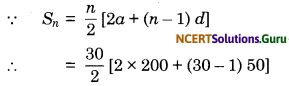= 15 (400 + 29 × 50)
= 15 (400 + 1450) = 15 × 1850.
= 27750
So, a delay of 30 days costs is ₹ 27750

Question 5.
A sum of ₹ 700 is to be used to give seven cash prizes to students of a school for their overall academic performance. If each prize is ₹ 20 less than its preceding prize, find the value of each of the prizes.
Solution.
Suppose, the respective prizes are a + 60, a + 40, a + 20, a, a – 20, a – 40, a – 60
According to question,
a + 60 + a + 40 + a + 20 + a + a – 20 + a – 40 + a – 60 = 700
⇒ 7a = 700
⇒ a = $$\frac {700}{7}$$ = 100
Hence, the seven prizes are 100 + 60, 100 + 40, 100 + 20, 100, 100 – 20, 100 – 40, 100 – 60 .’i.e., 160, 140, 120, 100, 80, 60, 40 .Question 6.
Two APs have the same common difference. The difference between their 100th terms is 100, what is the difference between their 1000th terms?
Solution.
Let the Two APs a1, a2, a3…….an and b1, b2, b3, …. bn
Also, let d be the same common difference of two APs. then
the nth term of first AP
On = a1 + (n-1)d and
the nth term of second AP
bn = b1 + (n – 1)d
Now, an – bn = [a1 + (n – 1)d] – [b1 + (n – 1)d]
⇒ an – bn = a1 – by for all n ∈ N
⇒ a100 – b100 = a1 – b1 = 100 (Given)
∴ a1000 – b1000 = a1 – b1
⇒ a1000 – b1000 = 100 [∵ a1 – b1 = 100]
Hence, the difference between their 1000th terms is also 100 for all n ∈ N.Question 7.
Have many three digit numbers are divisible by 7?
Solution.
We know that, 105 is the first and 994 is the last 3 digit number divisible by 7. Thus, we have to determine the number of terms in the list 105, 112, 119, …, 994.
Clearly, the successive difference of terms is constant with common difference
d = 112 – 105 = 7
So, it forms an AP.
Let there be n terms, in the AP, then nth term = 994
∵ an = a + (n – 1)d
⇒ 105 + (n – 1)7 = 994
⇒ 7(n – 1) = 994 – 105
⇒ 7(n – 1) = 889
⇒ n – 1 = $$\frac {889}{7}$$
= 127
⇒ n = 127 + 1 = 128
So, there are 128 numbers of three digit which are divisible by 7.Question 8.
How many multiple of 4 lie between 10 and 250?
Solution.
We see that 12 is the first integer between 10 and 250, which is a multiple of 4. Also, when we divide 250 by 4, the remainder is 2. Therefore, 250 – 2 = 248 is the greatest integer divisible by 4 and lying between 10 and 250. Thus, we have to find the number of terms in an AP whose first term = 12, last term = 248 and common difference = 4.
Let the n term of the AP, is
an = 248
= 12 + (n – 1) 4 = 248
[∵ an= a + (n – 1)d]
⇒ 4(n – 1) = 248 – 12
⇒ 4(n – 1) = 236
⇒ n – 1 = $$\frac {236}{4}$$ = 59
⇒ n = 59 + 1 = 60
Hence, there are 60 multiples of 4 lie between 10 and 250.

### Arithmetic Progressions Class 10 Extra Questions Long answer Type

Question 1.
A spiral is made up of successive semicircles with centres alternately at A and B, starting with centre at A, of radii 0.5 cm, 1.0 cm, 1.5 cm, 2.0 cm, …. as shown in figure. What is the total length of such a spiral made up of thirteen consecutive semi-circles?
[Take, π = $$\frac {22}{7}$$ ]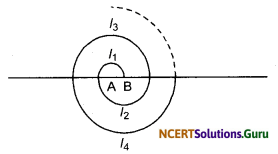Solution.
Length of spiral made up of thirteen consecutive semi-circles
= (π × 0.5 + π × 1.0 + π × 1.5 + π × 2.0 + … + π × 6.5)
= π × 0.5 (1 + 2 + 3 + … + 13)
which form an AP with first term, a = 1,
common difference, d = 2 – 1 = 1
and number of term, n = 13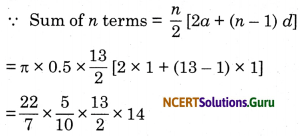= 143 cm.Question 2.
200 logs are stacked in the following manner: 20 logs in the bottom row, 19 in the next row, 18 in the row next to it and so on (see figure). In how many rows are the 200 logs placed and how many logs are in the top row?Solution.
Since, logs are stacked in each row form a series 20 + 19 + 18 + 17 + …. Clearly, it is an AP with first term, a = 20 and common difference, d = 19 – 20 = -1
Suppose, Sn = 200
∵ Sn = $$\frac {n}{2}$$[2a + (n – 1) d]
⇒ 200 = $$\frac {n}{2}$$[2 × 20 + (n – 1) (-1)]
⇒ 400 = n(40 – n + 1)
⇒ n2 – 41n + 400 = 0
⇒ n2 – 25n – 16n + 400 = 0
(By factorization method)
⇒ n(n-25) – 16 (n – 25) = 0
⇒ (n – 25) (n – 16) = 0
⇒ n = 16
or n = 25
Hence, the number of rows is either 25 or 16.
When n = 16, tn = a + (n – 1)
= 20 + (16 – 1) (-1)
= 20 – 15
= 5
When n = 25,
tn = a+ (n – l) d
= 20 + (25 – 1) (-1)
20 – 24 = -4 (Not possible)
Hence, the number of row is 16 and number of logs in the top row = 5.Question 3.
In a potato race, a bucket is placed at the starting point, which is 5 m from the first potato, and the other potatoes are placed 3 m apart in a straight line. There are ten potatoes in lines (see figure) A competitor starts from the bucket, picks up the nearest potato, runs back with it, drops it in the bucket, runs back to pick up the next potato, runs to the bucket to drop it in, and she continues in the same way until all the potatoes are in the bucket. What is the total distance the competitor has to run?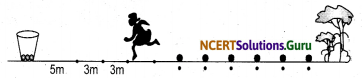Solution.
According to question, a competitor pick up the Ist potato, second potato, third potato, fourth potato ….
The distances sum by competitor are 2 × 5, 2 × (5 + 3), 2 × (5 + 3 + 3), 2 × (5 + 3 + 3 + 3) i.e., 10, 16, 22, 28, …..
Clearly, it is an AP with first term, a = 10
and common difference, d = 16 – 10 = 6
∵ The sum of n terms,
Sn = $$\frac {n}{2}$$[2a + (n – 1) d]
∴ The sum of 10 terms,
S10 = $$\frac {10}{2}$$[2 × 10 + (10 – 1) × 6]
[∵ n = 10 (given)]
= 5 (20 + 54)
= 5 × 74
= 370
Hence, the total distance the competitor has to run = 370 m.error: Content is protected !!